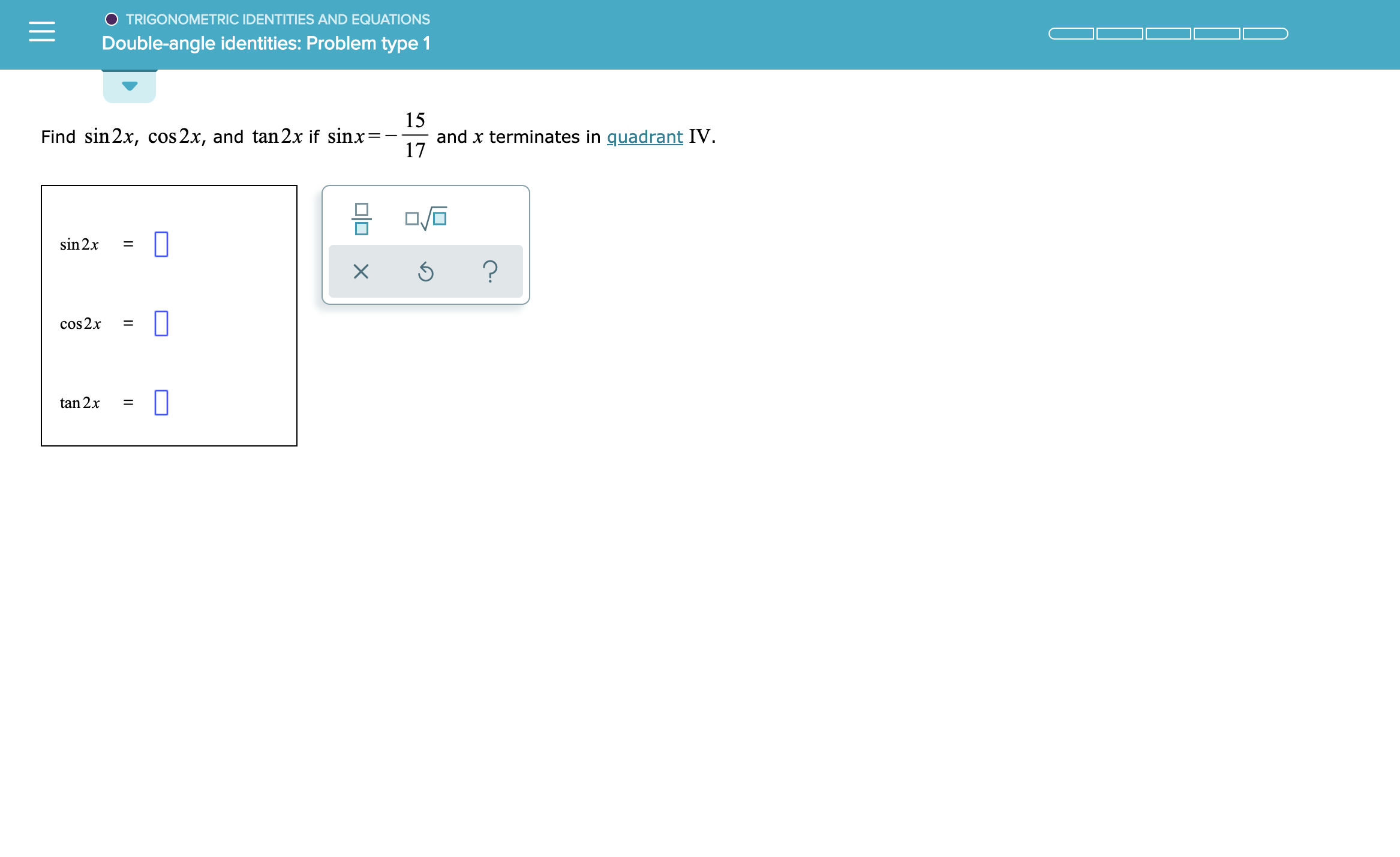# O TRIGONOMETRIC IDENTITIES AND EQUATIONSDouble-angle identities: Problem type 115and x terminates in quadrant IV17Find sin 2x, cos 2x, and tan2x if sinx=sin 2x?cos2x=tan 2x

Question
3 views

See attachmenthelp_outlineImage TranscriptioncloseO TRIGONOMETRIC IDENTITIES AND EQUATIONS Double-angle identities: Problem type 1 15 and x terminates in quadrant IV 17 Find sin 2x, cos 2x, and tan2x if sinx= sin 2x ? cos2x = tan 2x fullscreen
check_circle

Step 1

Given

Step 2

So, value of cosx is given by formula

Step 3

Value of sin2x is giv...

### Want to see the full answer?

See Solution

#### Want to see this answer and more?

Solutions are written by subject experts who are available 24/7. Questions are typically answered within 1 hour.*

See Solution
*Response times may vary by subject and question.
Tagged in

### Other